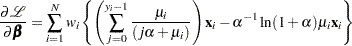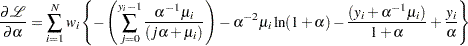Negative Binomial Regression

The Poisson regression model can be generalized by introducing an unobserved heterogeneity term for observation. Thus, the individuals are assumed to differ randomly in a manner that is not fully accounted for by the observed covariates. This is formulated as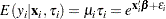where the unobserved heterogeneity term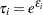is independent of the vector of regressors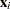. Then the distribution of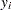conditional onand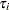is Poisson with conditional mean and conditional variance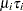: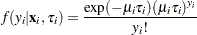Let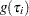be the probability density function of. Then, the distribution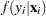(no longer conditional on) is obtained by integrating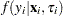with respect to: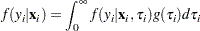An analytical solution to this integral exists whenis assumed to follow a gamma distribution. This solution is the negative binomial distribution. When the model contains a constant term, it is necessary to assume that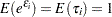, in order to identify the mean of the distribution. Thus, it is assumed thatfollows a gamma(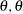) distribution with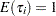and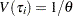,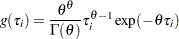where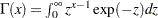is the gamma function and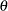is a positive parameter. Then, the density ofgiven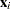is derived as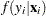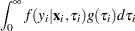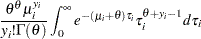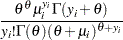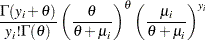Making the substitution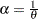(
), the negative binomial distribution can then be rewritten as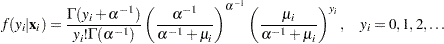Thus, the negative binomial distribution is derived as a gamma mixture of Poisson random variables. It has conditional mean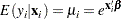and conditional variance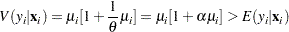The conditional variance of the negative binomial distribution exceeds the conditional mean. Overdispersion results from neglected unobserved heterogeneity. The negative binomial model with variance function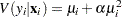, which is quadratic in the mean, is referred to as the NEGBIN2 model (Cameron and Trivedi 1986). To estimate this model, specify DIST=NEGBIN(p=2) in the MODEL statement. The Poisson distribution is a special case of the negative binomial distribution where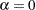. A test of the Poisson distribution can be carried out by testing the hypothesis that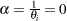. A Wald test of this hypothesis is provided (it is the reportedstatistic for the estimated
in the negative binomial model).

The log-likelihood function of the negative binomial regression model (NEGBIN2) is given by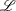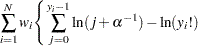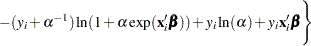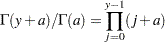if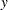is an integer. See Poisson Regression for the definition of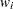.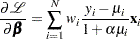and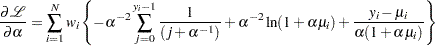Cameron and Trivedi (1986) consider a general class of negative binomial models with mean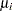and variance function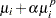. The NEGBIN2 model, with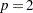, is the standard formulation of the negative binomial model. Models with other values of,
, have the same densityexcept that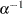is replaced everywhere by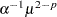. The negative binomial model NEGBIN1, which sets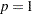, has variance function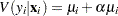, which is linear in the mean. To estimate this model, specify DIST=NEGBIN(p=1) in the MODEL statement.

The log-likelihood function of the NEGBIN1 regression model is given by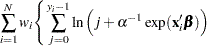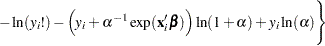See the section Poisson Regression for the definition of.